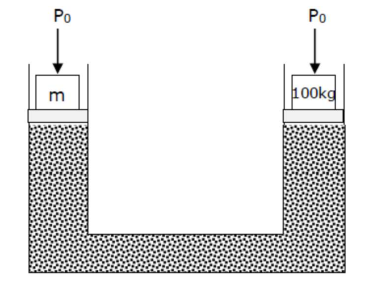# A hydraulic press can lift 100 kg when a mass ' m ' is placed on the smaller piston.Question:

A hydraulic press can lift $100 \mathrm{~kg}$ when a mass ' $\mathrm{m}$ ' is placed on the smaller piston.

It can lift______ $\mathrm{kg}$ when the diameter of the larger piston is increased by 4 times and that of the smaller piston is decreased by 4 times keeping the same mass ' $m$ ' on the smaller piston.

Solution:

$(25600)$Atmospheric pressure $P_{0}$ will be acting on both the limbs of hydraulic lift.

Applying pascal's law for same liquid level

$\Rightarrow \mathrm{P}_{0}+\frac{\mathrm{mg}}{\mathrm{A}_{1}}=\mathrm{P}_{0}+\frac{(100) \mathrm{g}}{\mathrm{A}_{2}}$

$\Rightarrow \frac{\mathrm{Mg}}{\mathrm{A}_{1}}=\frac{(100) \mathrm{g}}{\mathrm{A}_{2}} \Rightarrow \frac{\mathrm{m}}{100}=\frac{\mathrm{A}_{1}}{\mathrm{~A}_{2}} \ldots(1)$

Diameter of piston on side of $100 \mathrm{~kg}$ is increased by 4 times so new area $=16 \mathrm{~A}_{2}$

Diameter of piston on side of $(\mathrm{m}) \mathrm{kg}$ is decreasing

$A_{1}=\frac{A_{1}}{16}$

(In order to increasing weight lifting capacity, diameter of smaller piston must be

reduced)

Again, $\frac{m g}{\left(\frac{A_{1}}{16}\right)}=\frac{M^{\prime} g}{16 A_{2}} \Rightarrow \frac{256 m}{M^{\prime}}=\frac{A_{1}}{A_{2}}$

From equation $(1)=\frac{256 \mathrm{~m}}{\mathrm{M}^{\prime}}=\frac{\mathrm{m}}{100} \Rightarrow \therefore \mathrm{M}^{\prime}=25600 \mathrm{~kg}$